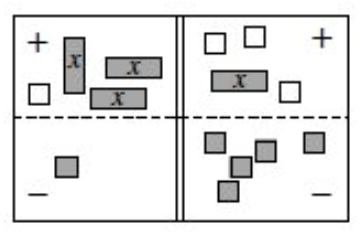### Home > AC > Chapter 2 > Lesson 2.2.2 > Problem2-104

2-104.

Write the equation represented in the equation mat below.1. Simplify both sides as much as possible, and then solve for $x$.

Write the equation. $3x+(−1)−1=x+(−3)−5$

One strategy is to flip all of the subtraction terms into the addition area and then simplify.

$3x+(−1)+(−1)=x+(−3)+(−5)$
$3x+(−2)=x+(−8)$

$x=−3$

1. Evaluate both the left side and the right side using your solution from part (a). Remember that if your solution is correct, both sides should have the same value.

Rewrite the equation with the known value of $x$ substituted in for $x$.